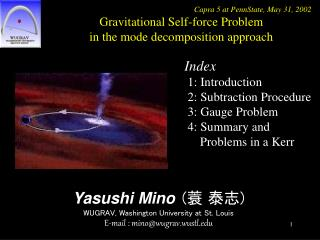DownloadDownload PresentationYasushi Mino ( 蓑 泰志 ) WUGRAV, Washington University at St. Louis E-mail : mino@wugrav.wustl

# Yasushi Mino ( 蓑 泰志 ) WUGRAV, Washington University at St. Louis E-mail : mino@wugrav.wustl

Download Presentation## Yasushi Mino ( 蓑 泰志 ) WUGRAV, Washington University at St. Louis E-mail : mino@wugrav.wustl

- - - - - - - - - - - - - - - - - - - - - - - - - - - E N D - - - - - - - - - - - - - - - - - - - - - - - - - - -
##### Presentation Transcript

1. Capra 5 at PennState, May 31, 2002 Gravitational Self-force Problem in the mode decomposition approach Index 1: Introduction 2: Subtraction Procedure 3: Gauge Problem 4: Summary and Problems in a Kerr Yasushi Mino(蓑 泰志) WUGRAV, Washington University at St. Louis E-mail : mino@wugrav.wustl.edu

2. Capra 5 at PennState, May 31, 2002 Gravitational Self-force Problem in the mode decomposition approach 1: Introduction LISA primary target A compact object (~1M) is inspiralling into a super-massive blackhole (~10^6M). *extreme mass ratio *eccentric orbit *relativistic motion We need to know what gravitational waves are expected to be detected.

3. Capra 5 at PennState, May 31, 2002 Gravitational Self-force Problem in the mode decomposition approach We use a perturbation analysis. A supermassive blackhole is taken to be a background (Kerr blackhole), and we deal with a spiralling star as a source of the perturbation. We consider the evolution of the orbit under the radiation reaction.

4. Capra 5 at PennState, May 31, 2002 Gravitational Self-force Problem in the mode decomposition approach 2: Subtraction Procedure : Full Metric perturbation : Direct Part of perturbation *One can take the limit only after the subtraction.

5. Capra 5 at PennState, May 31, 2002 Gravitational Self-force Problem in the mode decomposition approach We replace the divergence by the sum of infinite harmonic series where each term is finite, and subtract the direct part term-by-term.

6. Capra 5 at PennState, May 31, 2002 Gravitational Self-force Problem in the mode decomposition approach • We determine the S part by using a local analysis. • Calculation procedure: • evaluate the direct part by the local coordinate expansion method • apply the mode decomposition (mode-sum) formula Reference: L.Barack, Y.Mino, H.Nakano, A.Ori, M.Sasaki, gr-qc/0111001 Y.Mino, H.Nakano, M.Sasaki, gr-qc/0111074 L.Barack, A.Ori, gr-qc/0204093

7. Capra 5 at PennState, May 31, 2002 Gravitational Self-force Problem in the mode decomposition approach 3: Gauge Problem - Finite Algebraically Local Gauge Condition - Force is a gauge dependent variable. We must adopt a gauge condition in the regularization calculation in a consistent manner. If we ignore this condition, we may have a residual divergence. The regularization scheme is defined in the harmonic gauge, therefore, we need a formula to calculate the metric perturbation in the harmonic gauge condition.

8. Capra 5 at PennState, May 31, 2002 Gravitational Self-force Problem in the mode decomposition approach The self-force is interpreted as a force by the R-part metric perturbation. At this stage, we can add a finite gauge transformation. (The gauge transformation must be defined in a whole geometry.)

9. Capra 5 at PennState, May 31, 2002 Gravitational Self-force Problem in the mode decomposition approach I found a nice choice of the finite gauge! We use the algebraic gauge transformation given in the Zerilli’s paper to R-part of the metric perturbation in the harmonic gauge.

10. Capra 5 at PennState, May 31, 2002 Gravitational Self-force Problem in the mode decomposition approach Comparison with the master variable- regularization For this scheme, we need to evaluate non-vanishing part of the S-part in the Regge-Wheeler gauge. ? In the present scheme, we only need the non-vanishing part of the S-part in the harmonic gauge.

11. Capra 5 at PennState, May 31, 2002 Gravitational Self-force Problem in the mode decomposition approach • Calculation procedure of the direct part: • the local coordinate expansion of the direct part in the harmonic gauge • apply the mode decomposition formula • calculate the gauge transformation to the Regge-Wheeler gauge • evaluate the direct part of the force in the Regge-Wheeler gauge

12. Capra 5 at PennState, May 31, 2002 Gravitational Self-force Problem in the mode decomposition approach 1) Local coordinate expansion in the harmonic gauge

13. Capra 5 at PennState, May 31, 2002 Gravitational Self-force Problem in the mode decomposition approach 2) Mode decomposition of the metric the harmonic gauge

14. Capra 5 at PennState, May 31, 2002 Gravitational Self-force Problem in the mode decomposition approach 4) Evaluation of the force in the Regge-Wheeler gauge

15. Capra 5 at PennState, May 31, 2002 Gravitational Self-force Problem in the mode decomposition approach The direct part of the force in the Regge-Wheeler gauge

16. Capra 5 at PennState, May 31, 2002 Gravitational Self-force Problem in the mode decomposition approach 4: Summary and Problems in Kerr We consider a modified regularization for the self-force. The gauge condition is defined to be Regge-Wheeler one in the R-part of the metric perturbation.

17. Capra 5 at PennState, May 31, 2002 Gravitational Self-force Problem in the mode decomposition approach We do not have a full metric perturbation formalism in the ‘Regge-Wheeler’gauge in a Kerr, but, it may be possible in a renormalized perturbative approach. In the electromagnetic case, we have a systematic expansion with respect to the spin parameter (a), which has an infinite convergence radius. The gravitational case is in progress.